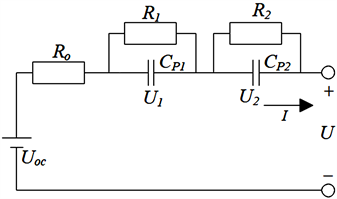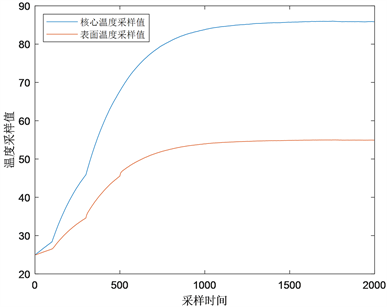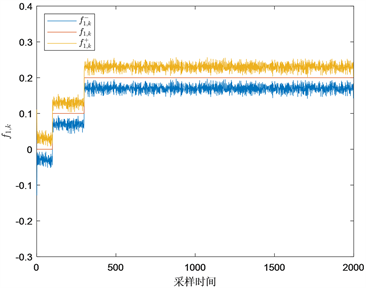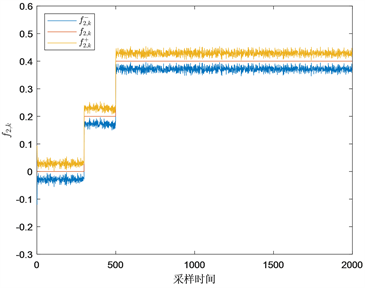#### 期刊菜单

Thermal Fault Diagnosis of Li-Ion Battery Actuator Based on Temperature Sampling Data
DOI: 10.12677/JEE.2021.92007, PDF, HTML, XML, 下载: 228  浏览: 464

Abstract: A fault diagnosis method based on set membership filtering is proposed for thermal fault of Li-ion battery. Through constructing electrothermal coupled model, based on the battery temperature sampling data, the Zonotopic Kalman filter algorithm is used to analyze the sampling data to obtain the interval of fault estimation, so as to realize the thermal fault diagnosis of Li-ion battery actuator.

1. 引言

2. 模型建立Figure 1. Schematic diagram of second-order Thevenin model

$\begin{array}{l}U={U}_{oc}-{R}_{o}I-{U}_{1}-{U}_{2}\hfill \\ \left\{\begin{array}{l}{\stackrel{˙}{U}}_{1}=-\frac{1}{{R}_{1}{C}_{p1}}{U}_{1}+\frac{1}{{C}_{p1}}I\\ {\stackrel{˙}{U}}_{2}=-\frac{1}{{R}_{2}{C}_{p2}}{U}_{2}+\frac{1}{{C}_{p2}}I\end{array}\hfill \end{array}$ (1)

$\left\{\begin{array}{l}{C}_{c}{\stackrel{˙}{T}}_{c}={Q}_{gen}+\frac{{T}_{s}-{T}_{c}}{{R}_{c}}\hfill \\ {C}_{s}{\stackrel{˙}{T}}_{\text{s}}=\frac{{T}_{e}-{T}_{s}}{{R}_{u}}-\frac{{T}_{s}-{T}_{c}}{{R}_{c}}\hfill \end{array}$ (2)

${Q}_{gen}=I\left({U}_{oc}-U\right)=I\left({R}_{o}I+{U}_{1}+{U}_{2}\right)$ (3)

$\left\{\begin{array}{l}{x}_{k+1}=A{x}_{k}+B{u}_{k}+{D}_{1}{w}_{k}\hfill \\ {y}_{k}=C{x}_{k}+{D}_{2}{v}_{k}\hfill \end{array}$ (4)

$A=\left[\begin{array}{cc}1-\frac{\text{Δ}t}{{R}_{c}{C}_{c}}& \frac{\text{Δ}t}{{R}_{c}{C}_{c}}\\ \frac{\text{Δ}t}{{R}_{c}{C}_{s}}& 1-\frac{\text{Δ}t}{{R}_{c}{C}_{s}}-\frac{\text{Δ}t}{{R}_{u}{C}_{s}}\end{array}\right],B=\left[\begin{array}{cc}\frac{\text{Δ}t}{{C}_{c}}& 0\\ 0& \frac{\text{Δ}t}{{R}_{u}{C}_{s}}\end{array}\right],C=\left[\begin{array}{cc}1& 0\\ 0& 1\end{array}\right],{D}_{1}={D}_{2}=\left[\begin{array}{cc}1& 0\\ 0& 1\end{array}\right]$

3. 集员滤波方法

4. 基于ZKF的锂电池执行器热故障诊断

$\left\{\begin{array}{l}{x}_{k+1}=A{x}_{k}+B{u}_{k}+{f}_{k}+{D}_{1}{w}_{k}\hfill \\ {y}_{k}=C{x}_{k}+{D}_{2}{v}_{k}\hfill \end{array}$ (5)

${\stackrel{¯}{x}}_{k}=\left[\begin{array}{c}{x}_{k}\\ {f}_{k-1}\end{array}\right]$ (6)

$\left\{\begin{array}{l}E{\stackrel{¯}{x}}_{k+1}=\stackrel{¯}{A}{\stackrel{¯}{x}}_{k}+\stackrel{¯}{B}{u}_{k}+{\stackrel{¯}{D}}_{1}{w}_{k}\hfill \\ {y}_{k}=\stackrel{¯}{C}{\stackrel{¯}{x}}_{k}+{D}_{2}{v}_{k}\hfill \end{array}$ (7)

$\begin{array}{l}E=\left[\begin{array}{cccc}1& 0& -1& 0\\ 0& 1& 0& -1\\ 0& 0& 0& 0\\ 0& 0& 0& 0\end{array}\right],\stackrel{¯}{A}=\left[\begin{array}{cccc}1-\frac{\text{Δ}t}{{R}_{c}{C}_{c}}& \frac{\text{Δ}t}{{R}_{c}{C}_{c}}& 0& 0\\ \frac{\text{Δ}t}{{R}_{c}{C}_{s}}& 1-\frac{\text{Δ}t}{{R}_{c}{C}_{s}}-\frac{\text{Δ}t}{{R}_{u}{C}_{s}}& 0& 0\\ 0& 0& 0& 0\\ 0& 0& 0& 0\end{array}\right],\stackrel{¯}{B}=\left[\begin{array}{cc}\frac{\text{Δ}t}{{C}_{c}}& 0\\ 0& \frac{\text{Δ}t}{{R}_{u}{C}_{s}}\\ 0& 0\\ 0& 0\end{array}\right],\\ {\stackrel{¯}{D}}_{1}=\left[\begin{array}{cc}1& 0\\ 0& 1\\ 0& 0\\ 0& 0\end{array}\right],\stackrel{¯}{C}=\left[\begin{array}{cccc}1& 0& 0& 0\\ 0& 1& 0& 0\end{array}\right]\end{array}$

$\begin{array}{l}{w}_{k}\in \mathcal{W}=〈0,W〉\\ {v}_{k}\in \mathcal{V}=〈0,V〉\end{array}$ (8)

${\stackrel{^}{\stackrel{¯}{\chi }}}_{k+1}=〈{\stackrel{^}{\stackrel{¯}{p}}}_{k+1},{\stackrel{^}{\stackrel{¯}{G}}}_{k+1}〉$ (9)

${\stackrel{^}{\stackrel{¯}{p}}}_{k+1}=T\stackrel{¯}{A}{\stackrel{¯}{p}}_{k}+T\stackrel{¯}{B}{u}_{k}+N{y}_{k+1}$ (10)

${\stackrel{^}{\stackrel{¯}{G}}}_{k+1}=\left[T\stackrel{¯}{A}{↓}_{re}{\stackrel{¯}{G}}_{k}\text{ }T{\stackrel{¯}{D}}_{1}W\text{}-N{D}_{2}V\right]$ (11)

$T={\text{Θ}}^{†}{\alpha }_{1}+S\text{Ψ}{\alpha }_{1},N={\text{Θ}}^{†}{\alpha }_{2}+S\text{Ψ}{\alpha }_{2}$ (12)

$\mathcal{Z}=〈\stackrel{¯}{p},\stackrel{¯}{G}〉\subseteq \stackrel{¯}{p}\oplus rs\left(\stackrel{¯}{G}\right){B}^{n}$ (13)

$rs\left(\stackrel{¯}{G}\right)=\left[\begin{array}{cccc}\underset{j=1}{\overset{s}{\sum }}|{\stackrel{¯}{G}}_{1,j}|& 0& \cdots & 0\\ 0& \underset{j=1}{\overset{s}{\sum }}|{\stackrel{¯}{G}}_{2,j}|& \cdots & 0\\ ⋮& ⋮& \ddots & ⋮\\ 0& 0& \cdots & \underset{j=1}{\overset{s}{\sum }}|{\stackrel{¯}{G}}_{n,j}|\end{array}\right]$ (14)

$\mathcal{Z}=〈\stackrel{¯}{p},\stackrel{¯}{G}〉\subseteq 〈\stackrel{¯}{p},{↓}_{re}\stackrel{¯}{G}〉$ (15)

${↓}_{re}\stackrel{¯}{G}=\left\{\begin{array}{ll}\stackrel{¯}{G},\hfill & \text{}s\le q\hfill \\ \left[\begin{array}{cc}{\stackrel{¯}{G}}_{>}& rs\left({\stackrel{¯}{G}}_{<}\right)\end{array}\right],\hfill & \text{}s>q\hfill \end{array}$ (16)

${\mathcal{S}}_{k+1}=\left\{{\stackrel{¯}{x}}_{k+1}\in {ℝ}^{n+s}:|\stackrel{¯}{C}{\stackrel{¯}{x}}_{k+1}-{y}_{k+1}|\le {D}_{2}\stackrel{˜}{v}\right\}$ (17)

${\stackrel{¯}{x}}_{k+1}\in {\stackrel{¯}{\chi }}_{k+1}=〈{\stackrel{¯}{p}}_{k+1},{\stackrel{¯}{G}}_{k+1}〉$ (18)

${\stackrel{¯}{p}}_{k+1}={\stackrel{^}{\stackrel{¯}{p}}}_{k+1}+{L}_{k+1}\left({y}_{k+1}-\stackrel{¯}{C}{\stackrel{^}{\stackrel{¯}{p}}}_{k+1}\right)$ (19)

${\stackrel{¯}{G}}_{k+1}=\left[\left({I}_{n+p}-{L}_{k+1}\stackrel{¯}{C}\right){\stackrel{^}{\stackrel{¯}{G}}}_{k+1}\text{ }{L}_{k+1}{D}_{v}\right]$ (20)

${L}_{k+1}={\stackrel{^}{\stackrel{¯}{G}}}_{k+1}{\stackrel{^}{\stackrel{¯}{G}}}_{k+1}^{\text{T}}{\stackrel{¯}{C}}^{\text{T}}{\left(\stackrel{¯}{C}{\stackrel{^}{\stackrel{¯}{G}}}_{k+1}{\stackrel{^}{\stackrel{¯}{G}}}_{k+1}^{\text{T}}{\stackrel{¯}{C}}^{\text{T}}+{D}_{v}{D}_{v}^{\text{T}}\right)}^{-1}$ (21)

$\left\{\begin{array}{l}{z}^{-}\left(i\right)=p\left(i\right)-\underset{j=1}{\overset{s}{\sum }}|G\left(i,j\right)|\text{ }\text{ },\text{ }i=1,\cdots ,n\\ {z}^{+}\left(i\right)=p\left(i\right)-\underset{j=1}{\overset{s}{\sum }}|G\left(i,j\right)|\text{ }\text{ },\text{ }i=1,\cdots ,n\end{array}$ (22)

$\left\{\begin{array}{l}{\stackrel{¯}{x}}_{k+1}^{+}\left(i\right)={\stackrel{¯}{p}}_{k+1}\left(i\right)+\underset{j=1}{\overset{q}{\sum }}|{\stackrel{¯}{G}}_{k+1}\left(i,j\right)|\text{ }\text{ },\text{\hspace{0.17em}}\text{\hspace{0.17em}}i=1,\cdots ,n+p\hfill \\ {\stackrel{¯}{x}}_{k+1}^{-}\left(i\right)={\stackrel{¯}{p}}_{k+1}\left(i\right)-\underset{j=1}{\overset{q}{\sum }}|{\stackrel{¯}{G}}_{k+1}\left(i,j\right)|\text{ }\text{ },\text{\hspace{0.17em}}\text{\hspace{0.17em}}i=1,\cdots ,n+p\hfill \end{array}$ (23)

$\begin{array}{l}{f}_{k}^{+}=\left[\begin{array}{cc}0& {I}_{p}\end{array}\right]{\stackrel{¯}{x}}_{k+1}^{+}\\ {f}_{k}^{-}=\left[\begin{array}{cc}0& {I}_{p}\end{array}\right]{\stackrel{¯}{x}}_{k+1}^{-}\end{array}$ (24)

5. 仿真示例Table 1. Main parameters of the electrothermal coupling model of lithium battery

${f}_{k}={\left[{f}_{1,k}\text{ }{f}_{2,k}\right]}^{\text{T}}=\left\{\begin{array}{l}{\left[\begin{array}{cc}0& 0\end{array}\right]}^{\text{T}},0\le k<100\hfill \\ {\left[\begin{array}{cc}0.1& 0\end{array}\right]}^{\text{T}},100\le k<300\hfill \\ {\left[\begin{array}{cc}0.2& 0.2\end{array}\right]}^{\text{T}},300\le k<500\hfill \\ {\left[\begin{array}{cc}0.2& 0.4\end{array}\right]}^{\text{T}},k\ge 500\hfill \end{array}$ (25)Figure 2. Core and surface temperature samples

$S=\left[\begin{array}{cccccc}1& 0& 0& 0& 0& 0\\ 0& 1& 0& 0& 0& 0\\ 0& 0& 1& 0& 0& 0\\ 0& 0& 0& 1& 0& 0\end{array}\right]$ (26)

$T=\left[\begin{array}{cccc}0& 0& 0& 0\\ 0& 0& 0& 0\\ -1& 0& 1& 0\\ 0& -1& 0& 1\end{array}\right],N=\left[\begin{array}{cc}1& 0\\ 0& 1\\ 1& 0\\ 0& 1\end{array}\right]$ (27)(a)(b)

Figure 3. Lithium battery thermal fault and its estimation intervals

6. 结论

NOTES

*通讯作者。

  陈锦攀, 赵鸿, 钟国彬, 等. 锂电池储能系统专利技术发展态势[J]. 储能科学与技术, 2019, 8(3): 602-608.  魏婧雯. 储能锂电池系统状态估计与热故障诊断研究[D]: [博士学位论文]. 合肥: 中国科学技术大学, 2019.  苏伟, 钟国彬, 沈佳妮, 等. 锂离子电池故障诊断技术进展[J]. 储能科学与技术, 2019, 8(2): 225-236.  王世繁, 罗杨, 董亮, 等. 二阶Thevenin锂电池等效模型参数离线辨识[J]. 电子设计工程, 2018, 26(9): 46-49, 54.  刘新天, 张胜, 何耀, 等. 基于简化可变参数热模型的锂电池内部温度估计[J]. 华南理工大学学报(自然科学版), 2019, 47(4): 44-52.  Richardson, R.R. and Howey, D.A. (2015) Sensorless Battery Internal Temperature Estimation Using a Kalman Filter with Impedance Measurement. IEEE Transactions on Sustainable Energy, 6, 1190-1199. https://doi.org/10.1109/TSTE.2015.2420375  汤文涛, 王振华, 王烨, 等. 基于未知输入集员滤波器的不确定系统故障诊断[J]. 自动化学报, 2018, 44(9): 1717-1724.